## A sports car has a mass of 1500 kg and accelerates at 5.0 m/s2. What is the magnitude of the force acting on the sports car?

Question

A sports car has a mass of 1500 kg and
accelerates at 5.0 m/s2. What is the magnitude of
the force acting on the sports car?

in progress 0
4 months 2021-09-03T18:56:16+00:00 2 Answers 33 views 0

## Answers ( )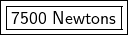Explanation:

Force is the product of mass and acceleration.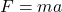The mass of the sports car is 1500 kilograms and the acceleration is 5.0 meters per square second.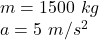Substitute the values into the formula.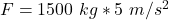Multiply.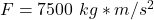• 1 kilogram meter per square second is equal to 1 Newton.
• Our answer is equal to 7500 Newtons.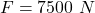The force acting on the sports car is 7500 Newtons.